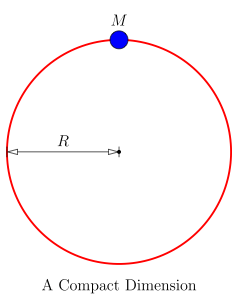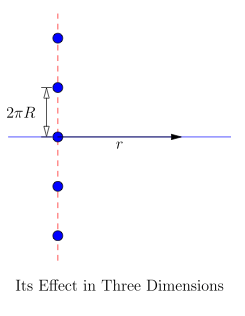# It Goes to Eleven

In Physics by Brian Koberlein17 Comments

When we look around the universe we see the usual dimensions of length, width and depth as we move forward through the dimension of time at the rate of one second per second, and it all makes sense to us. However string theory (or more properly its offspring, M-theory) says that although we may seem to live in these four dimensions, we actually live in a universe of eleven dimensions. According to string theory the universe only appears 4-dimensional because, like Edwin Abbott’s flatlanders, we are trapped on a “plane” of four dimensions. The reason we are trapped is that all the strings we are made of — the electron, neutron, and proton strings — are open strings. The ends of these strings can’t flop around freely, but instead must be fixed to a membrane surface or “brane”, and are thus only able to move freely along this brane, specifically our usual four dimensions. Closed strings on the other hand can flow freely through higher-dimensional space. In string theory gravity is made of such strings, which would explain why gravity is so much weaker than other forces such as electromagnetism. The basic idea is that all the other forces are trapped on the brane, and are thus very concentrated, whereas gravity is spread throughout all eleven dimensions, and so seems much weaker in our regular space-time.

Still, other than mathematical elegance, why invoke higher dimensions in the first place? If we can’t see them, and physical objects can’t travel through them, then aren’t they superfluous? Not quite. It turns out that even though we can’t see higher dimensions directly, might be able to detect them indirectly. To see how this might work, consider Newton’s theory of gravity in two dimensions.For flatland physicists, who live on the surface of a 2-dimensional plane. They look around and see that gravity behaves as it should for two dimensions. In two dimensions gravity is proportional to the inverse of distance, not the inverse square of the distance. If their plane existed in three dimensions, their gravity would be an inverse square force just like ours. But suppose that in their universe, the third dimension isn’t flat and open as it is in our universe, but instead loops back on itself. If they could travel in this third dimension, they would find that after a short journey they would find themselves back where they started. Their universe would be 3-dimensional, just very thin. Put another way, if they assumed the third dimension was flat, they would see images of their universe over and over again. In the first figure, I’ve drawn a simple diagram of a closed dimension. In the second figure I’ve drawn how the mass and all of its images would appear to our flatland physicists.In such a universe, the force of gravity would be the usual inverse square form with one catch. All the images of a mass would have the same gravitational attraction as real masses. Since the third dimension is compact, to find the force of gravity from a mass we would have to add up the effect of an infinite number of masses spread evenly along the third dimension. The result is a bit complicated in general, but if the third dimension is really thin, then at the scale of our physicists the mass would look like a continuous line of mass. It turns out that the gravitational field from a long line of mass is proportional to the inverse of your distance from the line, just as if space were two dimensional. On the other hand, if our flatland physicists measured gravity on a small scale (on the order of the size of the extra dimension) they would see the effect of the discrete mass images. In other words, they would see that gravity it is almost 2-dimensional, but has a small extra term due to the small compact dimension. Our 2-dimensional physicists would see 2-dimensional gravity in their everyday world, but they might detect the third dimension if they take a closer look at gravity on either very large or very small scales.

We can apply the same idea to our universe, and what we find is that if all the “extra” dimensions are compact we would never notice their presence unless we look very closely at things small scale, such as the strength of gravity. So far, experiments to detect deviations from Newtonian gravity at small scales haven’t found any higher-dimensional effect. What they have shown is that either the universe consists of only the four dimensions we see around us, or else all dimensions beyond those four must be very small, no more than about 10 microns, roughly one-tenth the width of a human hair.

1.cool

2.What would be the implications if the estra demnsions were infinitely small?

1.Author

If they were infinitely small they wouldn’t have any physical effect, so they might as well not exist.

3.10 microns is enough to get a pry bar in there . . . an 8 micron pry-bar . . . or better yet, point a laser beam into it and see where it comes out. Does “into it” have any meaning here?

1.Author

The problem is that your pry bar and even the laser beam are trapped in the usual 4 dimensions.

4.There is a simple description of eleven dimensions in Einsteins Intuition by Thad Roberts .

5.Why 3 dimensions or 4 dimensions or even 11 dimensions. An infinity of dimensions or even a “continuously dimensional” approach would allow a “continuously” variable gravity which also eliminate the need for dark matter or dark energy,

6.So I only understood about half of that (The easy half) and my question is about the following quote: “but they might detect the third dimension if they take a closer look at gravity on either very large or very small scales”

You talked about scientists applying this idea at small scales, but if this idea is applied to our universe at large scales, can dark matter or dark energy be implications of higher dimensions?

1.Author

Folks have looked at higher dimensional dark model ideas, but so far they don’t work very well.

7.“The reason we are trapped is that all the strings we are made of — the electron, neutron, and proton strings — are open strings. The ends of these strings can’t flop around freely, but instead must be fixed to a membrane surface or “brane”, and are thus only able to move freely along this brane, specifically our usual four dimensions.”,

What if we are made up of closed strings instead, whose ends are free to move through higher dimensional plane and this is continuously happening in an orderly fashion which would result in the phenomenon we call time?

8.Physics gives us formulas from an “explosive” expansion of the universe. Never was an “explosive” expansion, but, rather an expansion of the singularity. This universe exists inside the expanding universe. And, our physics cannot detect the expanding universe. It is, beyond our understanding. Studying this “spooky at a distance” can only lead to “ignorant zealous”.

9.Or is it, “zealous ignorance”?

10.Was “Spinal Tap” (motion picture) prescient? Or was a physicist on the writing team?
Just a random musing.

Rick J.
“Thankful for the rain after 57 days without it”
Portland, Oregon

11.John Birmingham’s The Axis of Time trilogy begins with experiments on these effects resulting in an accidental wormhole gobbling up an entire advanced Naval Taskforce in 2021 and transporting it back to 1942. Excellent 3 book series.

12.There is no such thing as gravity. Just electricity and magnetism.

1.Author